## research papersJOURNAL OFSYNCHROTRONRADIATION
ISSN: 1600-5775
Volume 26| Part 1| January 2019| Pages 152-158

## Ca and S K-edge XANES of CaS calculated by different methods: influence of full potential, core hole and Eu doping

aInstitute of Physics, Czech Academy of Sciences, Cukrovarnická 10, CZ-162 53 Prague, Czech Republic, bUniversity of West Bohemia, Univerzitní 8, CZ-306 14 Pilsen, Czech Republic, cDepartment of Physics, University of Lahore, Gujrat Campus, Gujrat, Pakistan, and dUniversité Grenoble Alpes, CNRS, Grenoble INP, Institut Néel, 38000 Grenoble, France
*Correspondence e-mail: sipr@fzu.cz

(Received 21 September 2018; accepted 14 November 2018)

Ca and S K-edge spectra of CaS are calculated by the full-potential Green's function multiple-scattering method, by the FLAPW method and by the finite-difference method. All three techniques lead to similar spectra. Some differences remain close to the edge, both when comparing different calculations with each other and when comparing the calculations with earlier experimental data. Here it is found that using the full potential does not lead to significant improvement over the atomic spheres approximation and that the effect of the core hole can be limited to the photoabsorbing atom alone. Doping CaS with Eu will not affect the Ca and S K-edge XANES of CaS significantly but may give rise to a pre-edge structure not present for clean CaS.

### 1. Introduction

Cubic calcium sulfide (CaS) is an important test material for studying the physical mechanism behind the processes in light-emitting diods (LEDs). This is especially true for CaS doped with rare-earth metals such as Eu (Tamura & Shibukawa, 1993; Zhang et al., 2005; Jia & Wang, 2007; Huang, 2015). A detailed understanding of electronic and spectroscopic properties of both clean and doped CaS is desirable. This applies also to the X-ray absorption spectra.

The accuracy of X-ray absorption near-edge structure (XANES) calculations has improved a lot during recent years. Among various factors affecting the accuracy of the calculation are the assumed shape of the potential (the full potential versus the muffin-tin potential) and the treatment of the core hole. One of the goals is to achieve a balance between the accuracy and computational demands. Therefore it is important to disentangle the physical effects from effects related to particular methods or codes.

The S K-edge XANES of CaS was investigated experimentally and theoretically in the past (Farrell et al., 2002; Kravtsova et al., 2004; Alonso Mori et al., 2009). High-resolution experimental data for both the Ca and S K-edge XANES were published in a recent study of Xu et al. (2013). They also performed calculations, either by the Green's function multiple-scattering method as implemented in the FDMNES code (Joly, 2015; Bunaˇu & Joly, 2009) and in the FEFF code (Rehr, 2013; Rehr et al., 2010) or by the finite-difference method (FDM) as implemented in the FDMNES code. The multiple-scattering calculations were performed relying on the muffin-tin approximation while the finite-difference method calculations were performed using a full potential (no shape approximation). Xu et al. (2013) obtained quite a good agreement between theory and experiment close to the edge. For the Ca K-edge, they found that the full-potential calculation led to a significantly better agreement between theory and experiment than the muffin-tin calculation. This can be considered as a surprise because it had been believed (based on previous experience) that full-potential effects are not very important for highly symmetric cubic structures (Huhne & Ebert, 1999). On the other hand, even the full-potential FDMNES calculations of Xu et al. (2013) failed to reproduced some small but well defined peaks occurring at 35 eV above the Ca K-edge and at 23 eV above the S K-edge. Green's function multiple-scattering calculations of Kravtsova et al. (2004) did not reproduce the peak at 23 eV above the S K-edge either. Xu et al. (2013) tentatively attributed this failure to radiation damage or to an unspecified charge transfer effect.

Given the importance of CaS for research on LED materials, it is desirable to understand its electronic structure correctly. In particular it would be interesting to understand more deeply the surprising findings which follow from the study of Xu et al. (the need for a full-potential treatment for the Ca K-edge together with the inability of both the multiple-scattering and FDM methods to reproduce some peaks relatively well above the edge). From a more general point of view, it is desirable to calculate XANES of CaS by different methods and to compare the results, so that differences which stem from using different calculational methods can be separated from differences which stem from using different physical approximations.

As mentioned in the beginning, the practical interest in CaS (and analogous sulfides) stems to a large extent from the fact that when doped with rare-earth atoms these systems form an important class of LED materials. The sulfides are particularly interesting because of their unusual dependence of the thermal quenching of the luminescence on the dopant (Dorenbos, 2005). To facilitate further experimental research on the electronic structure of doped sulfides, it would be instructive to investigate how the Ca and S K-edge XANES change if CaS is doped by a rare-earth metal such as Eu.

To achieve these goals, we performed XANES calculations for CaS using a fully relativistic full potential Green's function method as implemented in the SPRKKR code (Ebert, 2017; Ebert et al., 2011), using a full potential linearized augmented plane wave (FLAPW) method as implemented in the WIEN2K code (Blaha et al., 2001), and using an FDM as implemented in the FDMNES code (Joly, 2015; Bunaˇu & Joly, 2009). Apart from comparing the results of different codes and methods, we investigated the effect of the spherical potential approximation and of various technical procedures to include the core hole within the final-state approximation. We found that all three methods lead to similar spectra which are in a good agreement with experiment, even in those energy regions where problems were observed earlier. Nevertheless, some differences between theoretical and experimental spectra remain within the first 10 eV above the edge. We found that full-potential calculations do not lead to significant improvement over calculations performed within the atomic spheres approximation. The technical details about how the final-state approximation is implemented are not important. As concerns the effect of Eu doping, we found that it will not introduce significant changes in the Ca and S K-edge spectra.

### 2. Methods

We considered CaS in a B1 or rock salt structure (space group 225, Pearson symbol cF8), with lattice constant a0 = 5.595 Å. The Green's function or multiple-scattering calculations were performed using the SPRKKR code. The potential was either without any restriction (full potential mode) or subject to the atomic spheres approximation (ASA). The ASA is similar to the muffin-tin approximation but the atomic spheres are overlapping and fill the whole space so that there is no interstitial region. Even though formally less accurate than the muffin-tin approximation, the ASA often yields better results than the `pure' muffin-tin approximation; it has been suggested that the overlap between the spheres partially compensates for the defects of the spherical approximation (Zwierzycki & Andersen, 2009). To achieve a better filling of the space, we introduced empty spheres (or Voronoi polyhedra, in the full potential mode) at the interstitial sites. The SPRKKR calculations were performed in a fully relativistic mode (solving the Dirac equation), both in the reciprocal space and in the real space. For reciprocal-space calculations, the k-space integration was carried out via sampling on a regular k-mesh, using a grid of 45 × 45 × 45 points in the full Brillouin zone. When calculating the spectra in a real-space mode, a cluster of 123 atoms (radius of 8.7 Å) was employed, using the same self-consistent potential as in the reciprocal-space calculations. The maximum angular momentum used for the multipole expansion of the Green's function was ℓmax = 3. The full-potential mode relied on the shape functions, meaning that the full potential and ASA calculations were performed using the same formalism: in both cases, the same multiple-scattering equation is solved (Zeller, 1987; Huhne et al., 1998).

The WIEN2K calculations are based on the FLAPW method. The wavefunctions inside the muffin-tin spheres were expanded in spherical harmonics up to the maximum angular momentum ℓmax = 10. The wavefunctions in the interstitial region were expanded in plane waves, with the plane-wave cutoff chosen so that KRMT = 7 (RMT represents the smallest muffin-tin radius and K is the magnitude of the largest wavevector). The muffin-tin radii were 2.42 a.u. for Ca atoms and 2.31 a.u. for S atoms. The k-space integration was performed via a modified tetrahedron integration scheme, using 1000 k-points in the full Brillouin zone distributed according to the 10 × 10 × 10 Monkhorst–Pack grid.

The finite-difference method solves the Schrödinger equation by discretizing it over a grid of points in the volume where the calculation is made. The FDMNES code uses in fact a mixed approach: the wavefunctions are expanded in spherical waves within small spheres around the nuclei (similar as in the FLAPW method) while a standard FDM calculation is carried out in the interstitial region (Joly, 2001). The potential used for FDM calculations is self-consistent as concerns its spherical aspects: it is obtained so that first a self-consistent calculation using the multiple-scattering method within the muffin-tin approximation is performed and then one last iteration is performed to obtain the final potential without any shape restrictions (Bunaˇu & Joly, 2009). The spectra calculated by the FDMNES code for this study were obtained for clusters of 81 atoms (7 Å radii).

The calculations were carried out relying on the local density approximation (LDA) to treat the exchange and correlation effects. Additionally, the FDMNES calculations (working usually on a larger energy range) employ a real energy-dependent self-energy (Hedin & Lundqvist, 1969; Lee & Beni, 1977). One can thus expect slight shifts in the peak positions between the SPRKKR and WIEN2K calculations on the one hand and the FDMNES calculations on the other.

Calculations for Eu-doped CaS were performed using the WIEN2K code (FLAPW method). We employed a supercell of 64 atoms, with one Ca atom substituted by Eu. The muffin-tin radii were 2.50 a.u. for Ca atoms, 2.18 a.u. for S atoms and 2.50 a.u. for the Eu atom. The structure was relaxed (keeping the lattice constant intact) to account for the changes of the bond lengths around Eu. While the electronic structure of clean CaS can be described within the LDA, for Eu-doped CaS this would be inappropriate because of the strongly correlated electrons in the Eu orbitals. Therefore we used the LDA+U method as implemented in the WIEN2K code, with the effective on-site Coulomb interaction parameter U = 8.0 eV applied to the Eu 4f electrons. This value reproduces properly the splitting between the spin-up and spin-down electrons for the Eu2+ ion.

The raw theoretical spectra were convoluted by a Lorentzian with an energy-dependent full width at half-maximum set as w(E) = wcore + 0.05(EEedge). The constant part wcore accounts for the finite core hole lifetime [0.77 eV for the Ca K-edge and 0.52 eV for the S K-edge (Campbell & Papp, 2001)], the energy-dependent part accounts for the finite lifetime of the ejected photoelectron (Müller et al., 1982).

#### 2.1. The core hole treatment

The presence of the core hole can be a major factor in XANES. However, in the case of the K-edge spectra, where the electron is ejected into delocalized p states, its influence can often be described within the final-state approximation. It consists of evaluating the spectra for electron states which have relaxed to the presence of the core hole. Usually, the originally core electron is put into the valence band: technically this maintains the charge neutrality, physically this simulates the screening of the core hole.

For the SPRKKR calculations one can conveniently employ the embedded impurity formalism: first the electronic structure of the system without a core hole is calculated and then a Dyson equation is solved for an embedded impurity cluster centered around the atom with a core hole (Minár et al., 2006). The embedded cluster defines the region where the electronic structure is allowed to relax to the presence of the core hole; beyond it there is an unperturbed host. As we have formally an infinite system with just a single core hole, one does not need to care about possible interference of the holes.

The embedded cluster around the photoabsorbing atom contains 19 atoms in this study. That should be sufficient because the core hole is usually screened quite efficiently (Zeller, 1988). Indeed, one can make an approximation that the core hole does not influence the electronic structure of neighboring atoms at all, i.e. that its impact is limited just to the photoabsorbing atom. Such a situation can be modeled by the single-site impurity formalism: the system is treated as a substitutional alloy where the concentration of the normal (ground-state) atoms is 100% and the concentration of the photoabsorbing atoms (with the core hole) is 0%. Such a system can be treated by a computationally efficient coherent potential approximation (CPA). As the CPA gives access to type-resolved quantities, the XANES generated at the atom with the core hole can be calculated even if its concentration is formally zero.

Physically the single-site approach means that the influence of neighboring atoms on the atom with the core hole is taken into account while the influence of the core hole atom on the other atoms is neglected. In other words, the core hole is perfectly screened. The difference between the embedded-cluster and single-site approaches to the core hole could also be viewed as a difference between the time scales in which the electrons respond to the disturbance at the photoabsorbing atom. The embedded cluster approach assumes that the electrons adjust instantaneously to the perturbation. The single-site approach assumes that the electrons at neighboring atoms are latent with respect to the photoabsorption process.

For the WIEN2K calculations, we employed supercells of 64 atoms and assigned the core hole to one of them. This means that the electronic structure of the atoms which are close to the photoabsorbing atom is affected by the core hole and, at the same time, the large size of the supercell makes the interaction between two excited atoms practically negligible. Such an approach is equivalent to the embedded cluster approach described above. On the other hand, for the FDMNES calculations we accounted for the core hole at the photoabsorbing atom only (Bunaˇu & Joly, 2009). That is similar to the single-site CPA-like approach.

Apart from the final-state approximation, another commonly used procedure to deal with the core hole is the Slater transition-state method. It is similar to the final-state approximation, except that only a half of an electron is transferred from the core level to the valence band. The incentive for using this approach is that in this way one obtains a good estimate of transition energies.

CaS is an insulator, so the influence of the core hole should be more significant than in metals. To estimate the importance of the core hole effect and also to check the robustness of different computational approaches, we performed the calculations using the ground-state potential, the Slater transition-state approach and the final-state approximation.

### 3. Results: Ca and S K-edge XANES of CaS

#### 3.1. Comparison between theory and experiment

We begin by comparing calculations with experiment. This is shown in Fig. 1. The experiment is taken from the work of Xu et al. (2013). We compare it with three different full potential calculations. The core hole was included within the final-state approximation, as described in Section 2.1. Note that for the SPRKKR calculations the final-state approximation was implemented using the embedded impurity cluster method in this section.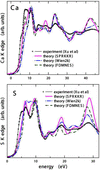Figure 1 Experimental Ca K-edge (upper panel) and S K-edge (lower panel) XANES of CaS (Xu et al., 2013) together with theoretical spectra obtained using the full-potential multiple-scattering method (SPRKKR code), the FLAPW method (WIEN2K code) and the full-potential FDM (FDMNES code).

One can see from Fig. 1that, even though all three calculations use essentially the same physical constraints (full potential, core hole via the final-state approximation), there are differences in the results. Some of these differences are not really significant. Others are more relevant, especially close to the edge (E < 10 eV), where none of the calculations reproduces the experiment very accurately. Nevertheless, the FDMNES calculation is closer to the experiment in this region than the SPRKKR or WIEN2K calculations. The reason for this is unclear.

#### 3.2. Comparing full-potential and ASA calculations

Fig. 2compares theoretical Ca and S K-edge XANES of CaS obtained for the full potential (FP) and for the spherical potential (ASA). The calculations were performed within the Green's function multiple-scattering formalism, by the SPRKKR code. The core hole was ignored. The spectra were calculated in the k-space. Additionally, the FP spectra were calculated also in the real space (123 atoms in the cluster, 8.7 Å radius). The experimental spectra of Xu et al. (2013) are shown as well so that one can better judge the significance of the changes induced by different theoretical modes.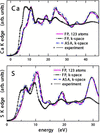Figure 2 Theoretical Ca K-edge (upper panel) and S K-edge (lower panel) XANES of CaS obtained in the full-potential (FP) mode either in the real space (for a cluster of 123 atoms) or in the reciprocal space, together with XANES obtained for a spherical potential (ASA) in the reciprocal space. The calculations were performed using the SPRKKR code; the core hole was not taken into account. The experimental XANES of Xu et al. (2013) is shown for comparison.

One can see that full-potential and ASA calculations lead to very similar spectra in our case, even in the near-edge region. This is different from the results of Xu et al. (2013): they found that for the Ca K-edge spectrum there is a small but significant difference between their Green's function multiple-scattering calculations carried out for a spherical muffin-tin potential and their FDM calculations carried out for a full potential.

For both the real-space and the reciprocal-space calculations, some technical issues have to be dealt with. For the real-space calculations, the question is whether the cluster is big enough. For the reciprocal-space calculations, the questions are about the k-mesh used for the Brillouin zone integration and about the convergence of the Ewald summation used to evaluate the structure constants. The real-space and the k-space spectra shown in Fig. 2are in a good agreement, confirming that in both cases the technical issues have been handled properly.

#### 3.3. Dealing with the core hole

Fig. 3shows how the spectra change if the core hole is switched on: one can see the results obtained within the final-state approximation (full core hole), within the Slater transition-state approximation (half core hole) and for the ground state (no core hole). The spectra were obtained using the SPRKKR code for a spherical ASA potential, using the embedded impurity cluster formalism. One can see that the core hole does not introduce new features in the spectra but it changes the intensities of peaks and shoulders. In particular, it enhances the features close to the absorption edge, in accordance with common experience (Weijs et al., 1990; Šipr et al., 1997; Šipr & Rocca, 2010).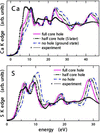Figure 3 Theoretical Ca K-edge (upper panel) and S K-edge (lower panel) XANES of CaS obtained when the core hole was accounted for via the final-state approximation, when the core hole was included via the Slater transition-state approach, and when it was ignored. The calculations were performed using the SPRKKR code in the ASA mode, via the embedded cluster formalism. The experimental XANES of Xu et al. (2013) is shown for comparison.

Comparison between spectra calculated using the embedded impurity cluster method and using the single-site impurity CPA-like approach is shown in Fig. 4. Again, the spectra were obtained for the spherical ASA potential. Physically, the difference between both processes is that for the embedded impurity cluster formalism the core hole affects not only the electronic structure of the photoabsorbing atom but also of a further 18 atoms in the two nearest coordination spheres, while for the single-site impurity formalism the core hole affects just the electronic structure of the photoabsorbing atom alone. It is obvious from Fig. 4that spectra obtained for both approaches lead to very similar spectra, meaning that the influence of the core hole on the neighboring atoms can be neglected.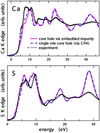Figure 4 Theoretical Ca K-edge (upper panel) and S K-edge (lower panel) XANES of CaS obtained when the core hole was accounted for via the final-state approximation relying either on the embedded impurity cluster formalism or on the single-site impurity approach. The calculations were performed using the SPRKKR code in the ASA mode. The experimental XANES of Xu et al. (2013) is shown for comparison.

#### 3.4. Influence of the Eu impurity

A lot of interest has been focused on properties of CaS (and other sulfides) doped with rare-earth metals. Fig. 5shows how Ca and S K-edge spectra of CaS change upon doping with Eu. We present the spectra generated at those atoms of given type which are closest to the Eu impurity because here the effect will be largest. It can be seen that the presence of Eu atoms does not change the spectra very much. Apart from the region at the very edge, no new features are introduced; just the intensities and positions of existing peaks and shoulders change slightly.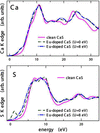Figure 5 Theoretical Ca K-edge (upper panel) and S K-edge (lower panel) XANES of Eu-doped CaS evaluated for those Ca or S atoms which are nearest to the Eu impurity. The calculations were performed using the WIEN2K code, either within the LDA framework (U = 0 eV) or using the LDA+U method (U = 8 eV). For the Ca K-edge, both spectral curves are practically identical. Results for clean CaS are shown for comparison.

Some pre-edge structure appears for both edges upon Eu doping; for the Ca K-edge this pre-peak occurs both for the LDA and LDA+U calculations while for the S K-edge it occurs only for the LDA case. This results from hybridization of Ca and S states with Eu states. The position of Eu states depends on U; it is thus not surprising that the appearance of the S K-edge pre-peak is strongly U-dependent.

Experimental XANES of doped CaS would be a superposition of spectra generated at all Ca or S atoms in the sample. Only a small fraction of them would occur near any Eu impurity. Given the overall similarity of the spectra for clean and doped CaS (Fig. 5), it is likely that the Eu doping will not affect the Ca and S K-edge XANES of CaS significantly, apart from a pre-edge structure which is not present for clean CaS but which might occur for doped CaS.

### 4. Discussion

Our goal was to check the robustness of XANES calculations on a case study of Ca and S K-edge spectra of CaS and to disentangle the effects of different physical approximations from the effects of using different computational techniques. We found that Green's function multiple-scattering calculations performed by the SPRKKR code, FLAPW calculations performed by the WIEN2K code, and FDM calculations performed by the FDMNES code yield similar results. The experimental spectra were reproduced quite well but there are some differences within the first 10 eV above the edge. Employing the full potential does not lead to significantly different spectra in comparison with the ASA. Including the core hole by the final-state approximation enhances some features close to the edge but generally does not lead to big changes of the spectra with respect to the ground-state calculations.

Earlier calculations of Xu et al. (2013) did not reproduce small but well defined peaks which appear in the experimental spectra at 35 eV above the Ca edge and at 23 eV above the S edge. The calculations of Kravtsova et al. (2004) did not reproduce the peak at 23 eV above the S K-edge either. Xu et al. (2013) tentatively attributed these peaks to radiation damage or to an unspecified charge transfer effect. However, all our calculations reproduce these peaks properly (cf. Fig. 1). It seems, therefore, that the failure of earlier calculations to obtain these peaks was due to some technical reasons. One of these might be that perhaps a too large broadening related to the finite photoelectron lifetime was applied. One should bear in mind that the values for the electron mean free path published by Müller et al. (1982) which are still commonly used were obtained by summarizing the trends for a large set of data for various materials; a large scatter of individual values around these estimates thus has to be anticipated (Gudat, 1974; Lindau & Spicer, 1974). Setting the photoelectron lifetime broadening empirically ad hoc probably still remains the most practical way to deal with this issue, despite promising results in the field of ab initio mean free path calculation (Kas et al., 2007; Chantler & Bourke, 2014; Emfietzoglou et al., 2017).

There is also a difference between our results and the results of Xu et al. (2013) concerning the importance of using the full potential. Our Green's function multiple-scattering calculations indicate that using the full potential does not significantly change the spectra with respect to the ASA results. The FDM calculations of Xu et al. (2013), on the other hand, suggest that using the full potential significantly improves the results within the first 10 eV above the edge, at least for the Ca K-edge spectrum. A possible explanation of this apparent contradiction could be that the spherical potential calculations performed via the SPRKKR code relied on the ASA while the spherical potential calculations of Xu et al. (2013) relied on the muffin-tin approximation. It has been argued that the ASA is better than the pure muffin-tin approximation (Zwierzycki & Andersen, 2009).

### 5. Conclusions

Theoretical Ca and S K-edge XANES spectra of CaS obtained by different full-potential methods (Green's function multiple-scattering, FLAPW, FDM) are mostly quite similar and in good agreement with experiment. In particular, all calculations reproduce the small but well defined peaks which appear in the experimental spectra at 35 eV above the Ca edge and at 23 eV above the S edge and which were not described by earlier calculations. Some differences between theory and experiment remain within the first 10 eV above the edge, where the finite-difference method (FDMNES code) leads to better agreement with experiment than the Green's function multiple-scattering method (SPRKKR code) or the FLAPW method (WIEN2K code).

Full-potential and atomic sphere approximation calculations performed within the Green's function multiple-scattering framework lead to very similar spectra. Including the core hole via the final-state approximation does not change the spectra significantly but it leads to enhancement of features close to the absorption edge. The influence of the core hole on the electronic structure of atoms next to the photoabsorbing atom can be neglected. Doping CaS with Eu will not affect the Ca and S K-edge XANES of CaS significantly.

### Funding information

Funding for this research was provided by: Grantová Agentura České Republiky (grant No. 17-14840 S); Ministerstvo Školství, Mládeže a Tělovýchovy (grant No. CZ.02.1.01/0.0/0.0/15_003/0000358).

### ReferencesJOURNAL OFSYNCHROTRONRADIATION